Articles

Parabolic SAR

Parabolic SAR is a technical analysis index developed by J. Welles Wilder that generally shows the price direction in the market. This indicator shows where the price of an asset will change direction, so it uses to open or close a position in a certain period.

Of course, most Parabolic SARs work when a market is trending. Based on the methods presented in this technical analysis, the trend direction should first be determined with Parabolic SAR. Then, using other indicators and analytical models, the strength of this trend to open or close a position should be examined.

When we look at a parabolic SAR on a graph, it seems more like the dots shown in parts of the chart. Parabolic SAR points consider as a positive signal if they are below the current price displayed. When the Parabolic SAR passes the current price and goes beyond it, it will be considered a Bearish signal. Signals use precisely to close or open a position.

How the Parabolic SAR formula works

Parabolic SAR is in the analytical chart of the price of an asset as points beside the price. In general, when the points are below the price line, we have a positive signal, and an upward trend is displayed. When the points are below the price line, we will have a bearish signal, and you should treat a specific asset based on it.

Shifting points indicates different signals for the asset being analyzed, and if used promptly, can yield considerable returns to investors. However, in markets with low volatility, this pattern does not work as well.Parabolic SAR can determine precisely when to open or close a position. Then you can decide when exactly to buy and sell an asset. However, if the market fluctuates sharply, Parabolic SAR also loses its effectiveness. If you can bring stop loss to SAR level, you can place stop-loss orders with Parabolic SAR.

What other indicators are compatible with Parabolic SAR?

In addition to Parabolic SAR, you should also use other analytical indicators like average directional index momentum indicators. Moving average and Candlestick are also analytical models that make a lot of money by working alongside Parabolic SAR. For example, if the price of an asset falls below the long-term moving average, the Parabolic SAR also shows a sell signal. If the price is higher than the moving average, a buy signal is also displayed.

How to Calculate the SAR Indicator

Parabolic SAR is calculated based on mathematical formulas and uses the maximum and minimum prices of an asset. The formula for this template is as follows:

1. Uptrend Parabolic SAR = Prior SAR + Prior AF (Prior EP – Prior SAR(
2. Downtrend Parabolic SAR = Prior SAR – Prior AF (Prior SAR – Prior EP(

EP is the highest price of an asset in a specified range in an uptrend routine or the lowest price in a downtrend routine.

AF shows the acceleration rate with an initial value of 0.02. You may ask why this is considered because the EP reaches a maximum of 0.2. An acceleration rate is required to adjust the trading method or the instrument used for the transaction.

Each calculation performed with the above formulas display as a dot on the graph. As an asset’s price line moves forward, points are also calculated online and placed on the price chart, and with these points, the price orientation can be determined.

Pros and Cons

The most significant advantage of the Parabolic SAR formula is that it can be used to determine the price direction. This index predicts fascinating results. Another positive point is that it determines the time of buying or selling relatively so that you can buy or sell simultaneously by suddenly changing the direction of the points produced by this formula.

Disadvantages of this formula include inefficiency when the price is highly volatile, or the price is not volatile at all. If the trend chart is insufficient, the points indicating the Parabolic SAR formula will go up and down and show the wrong signals. In this case, this formula can not be adhered to at all.

In this case, traders should use other analytical indicators to make more possible profits in flat markets.

Conclusion

Parabolic SAR is a technical analysis formula that determines the price orientation of an asset and shows you when to buy or sell an asset. Using this analysis and other indicators, you can profit from an investment in the Forex, cryptocurrency, or stock markets. However, this formula works best for volatile markets.

Excited
0
Happy
0
In Love
0
Not Sure
0
Silly
0

Articles

Articles

Articles

Articles

Articles

Articles

Bearish flag pattern

Articles
Next Article:
0 %
•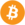Bitcoin (BTC) \$ 37,906.00
•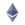Ethereum (ETH) \$ 2,541.85
•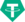Tether (USDT) \$ 1.00
•Binance Coin (BNB) \$ 384.45
•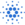Cardano (ADA) \$ 1.05
•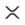XRP (XRP) \$ 0.612118
•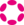Polkadot (DOT) \$ 18.37
•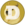Dogecoin (DOGE) \$ 0.142003
•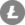Litecoin (LTC) \$ 109.26
•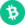Bitcoin Cash (BCH) \$ 295.39
•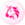Uniswap (UNI) \$ 10.59